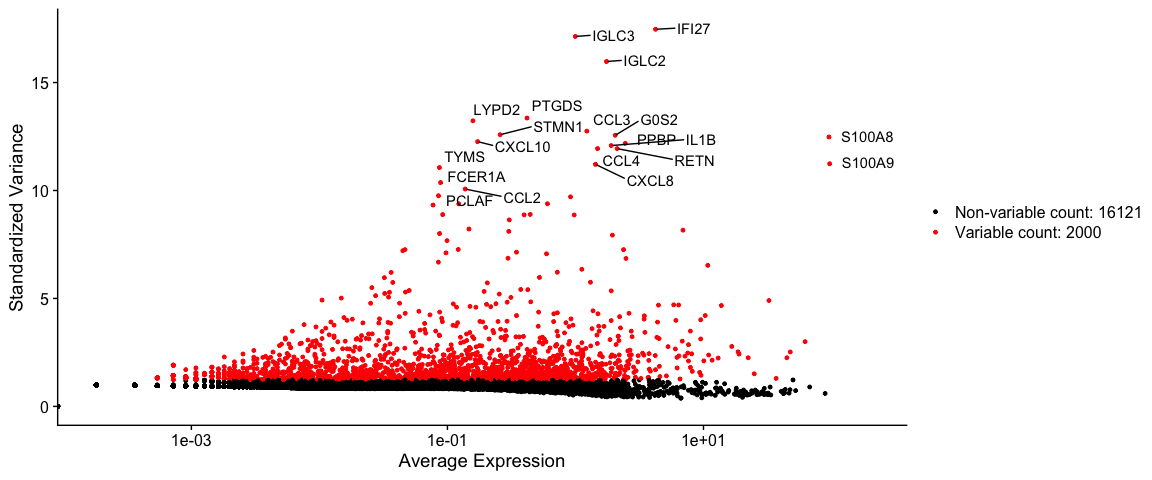# Dimensionality reduction

Paulo Czarnewski

## Data preparation

First, let’s load all necessary libraries and the QC-filtered dataset from the previous step.

``````suppressPackageStartupMessages({
library(Seurat)
library(cowplot)
library(ggplot2)
library(scran)
})

### Feature selection

Next, we first need to define which features/genes are important in our dataset to distinguish cell types. For this purpose, we need to find genes that are highly variable across cells, which in turn will also provide a good separation of the cell clusters.

``````suppressWarnings(suppressMessages(alldata <- FindVariableFeatures(alldata, selection.method = "vst",
nfeatures = 2000, verbose = FALSE, assay = "RNA")))

LabelPoints(plot = VariableFeaturePlot(alldata), points = top20, repel = TRUE)``````### Z-score transformation

Now that the data is prepared, we now proceed with PCA. Since each gene has a different expression level, it means that genes with higher expression values will naturally have higher variation that will be captured by PCA. This means that we need to somehow give each gene a similar weight when performing PCA (see below). The common practice is to center and scale each gene before performing PCA. This exact scaling is called Z-score normalization it is very useful for PCA, clustering and plotting heatmaps.
Additionally, we can use regression to remove any unwanted sources of variation from the dataset, such as `cell cycle`, `sequencing depth`, `percent mitocondria`. This is achieved by doing a generalized linear regression using these parameters as covariates in the model. Then the residuals of the model are taken as the “regressed data”. Although perhaps not in the best way, batch effect regression can also be done here.

``````alldata <- ScaleData(alldata, vars.to.regress = c("percent_mito", "nFeature_RNA"),
assay = "RNA")``````

## PCA

Performing PCA has many useful applications and interpretations, which much depends on the data used. In the case of life sciences, we want to segregate samples based on gene expression patterns in the data.

``alldata <- RunPCA(alldata, npcs = 50, verbose = F)``

We then plot the first principal components.

``````plot_grid(ncol = 3, DimPlot(alldata, reduction = "pca", group.by = "orig.ident",
dims = 1:2), DimPlot(alldata, reduction = "pca", group.by = "orig.ident", dims = 3:4),
DimPlot(alldata, reduction = "pca", group.by = "orig.ident", dims = 5:6))``````